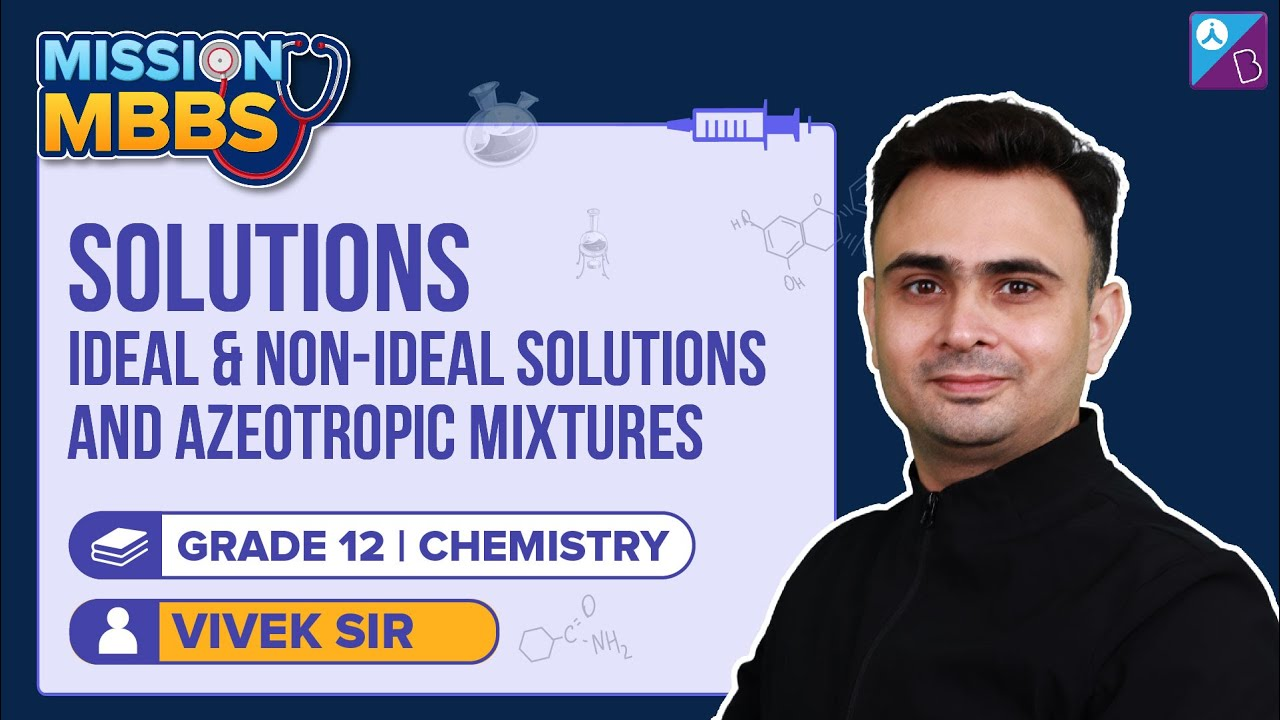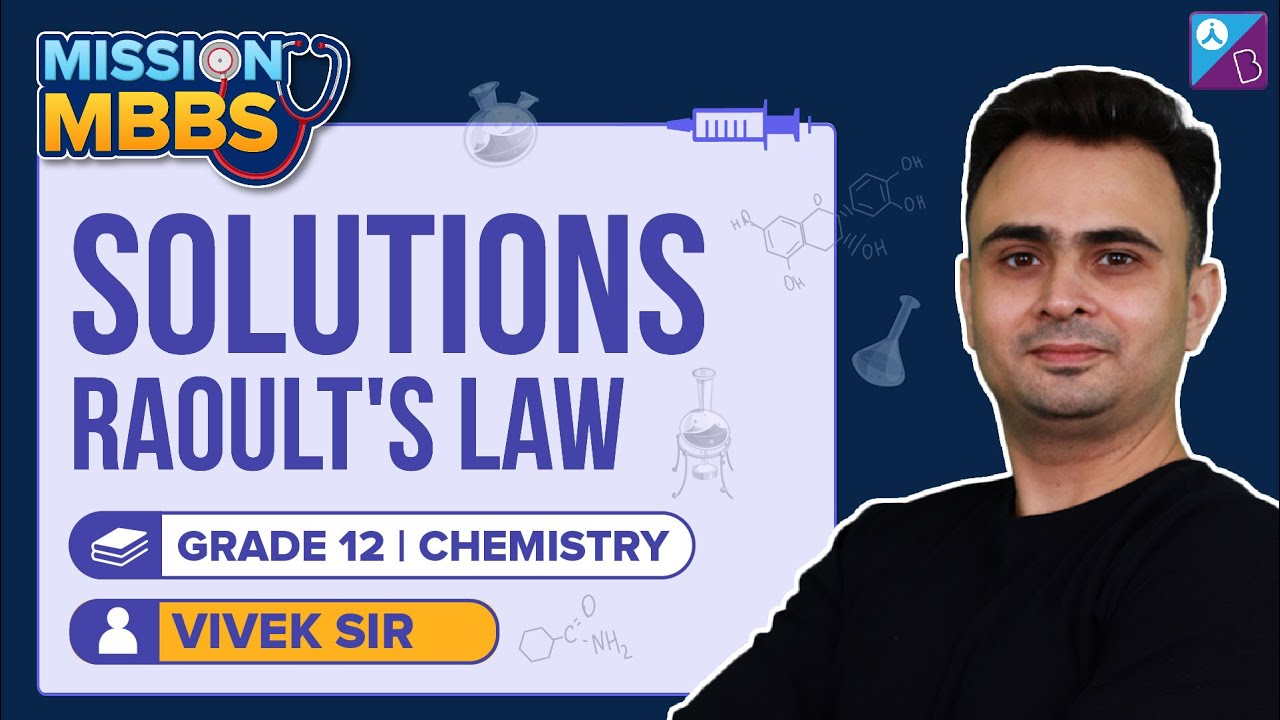Win up to 100% scholarship on Aakash BYJU'S JEE/NEET courses with ABNAT Win up to 100% scholarship on Aakash BYJU'S JEE/NEET courses with ABNAT

# Difference between Ideal Solution and Non-ideal Solution

Any homogeneous mixture of two or more components is known as a solution. The component having the highest quantity in a solution is known as the solvent, which determines the solution’s physical state. Other components are known as solutes. The solution with two components is known as a binary solution. The properties of a solution are expressed in terms of vapour pressure, boiling point, freezing point and other colligative properties.

The binary liquid in liquid solutions can be classified into two types; ideal and non-ideal solutions.Don’t miss: Answer Key of NEET 2022

## Ideal Solution

An ideal solution is a solution where the intermolecular interactions between solute-solute (A-A) and solvent-solvent (B-B) are similar to the interaction between solute-solvent (A-B). An ideal solution fulfils the following criteria:

• It obeys Raoult’s law for all the concentration and temperature ranges, which states that the partial vapour pressure of each component is proportional to the mole fraction of the component in a solution at a given temperature.
• The enthalpy of mixing is zero, i.e. ΔHmix = 0. It means that no heat is absorbed or released.
• The volume of mixing is zero, ΔVmix = 0. It means that the volume of the solution is equal to the sum of the volume of components.

The ideal solution is possible with components of the same size and polarity. There is no association, dissociation or reaction taking place between components. A perfect ideal solution is rare, but some solutions are near to the ideal solution. Examples are Benzene and toluene, hexane and heptane, bromoethane and chloroethane, chlorobenzene and bromobenzene, etc.

## Non-ideal Solution

When a solution does not obey Raoult’s law for all the concentration and temperature ranges, it is known as a non-ideal solution. A non-ideal solution may show positive or negative deviation from Raoult’s law. ΔHmix and ΔVmix for non-ideal solutions are not equal to zero.

a) Non-ideal solution showing positive deviation

Here the total vapour pressure is higher than that calculated from Raoult’s equation. The interaction between solute-solvent (A-B) is weaker than those of pure components (A-A or B-B). The ΔHmix and ΔVmix are positive. E.g. ethanol and acetone, carbon disulphide and acetone, acetone and benzene, etc.

b) Non-ideal solution showing negative deviation

Here the total vapour pressure is lower than that calculated from Raoult’s equation. The interaction between solute-solvent (A-B) is stronger than those of pure components (A-A or B-B). The ΔHmix and ΔVmix are negative. E.g. phenol and aniline, chloroform and acetone, etc.

## Difference between Ideal and Non-ideal Solution

The table below shows the main differences between Ideal and Non-ideal Solutions:

 Ideal Solution Non-ideal Solution Raoult’s Law They obey Raoult’s law They do not obey Raoult’s law Molecular Interactions Intermolecular interaction between solute and solvent is the same as that of pure components Intermolecular interaction between solute and solvent is weaker or stronger than that of between pure components Total Vapour Pressure The total vapour pressure is the same as predicted by Raoult’s law The total vapour pressure increases or decreases from the predicted value according to Raoult’s law Enthalpy of mixing No heat is released or absorbed, so the enthalpy of mixing is zero, ΔHmix = 0 Heat is either absorbed or released, so the enthalpy of mixing is either positive or negative, ΔHmix ≠ 0 Volume of mixing The total volume is equal to the sum of the volume of components (solute and solvent), so the volume of mixing is zero, ΔVmix = 0 The volume of mixing is not zero, ΔVmix ≠ 0. There is either expansion or contraction. Separation of components Components can be separated by fractional distillation Components can’t be separated in the pure form by fractional distillation Azeotrope formation Does not form an azeotrope Forms azeotrope mixture Examples Benzene and toluene, hexane and heptane, etc. All the dilute solutions nearly behave as an ideal solution Ethanol and acetone, carbon disulphide and acetone, phenol and aniline, chloroform and acetone, etc.

Explore all the NEET Chemistry Important Topics aligned with the updated NEET syllabus only at BYJU’S.

### Recommended Video:

Raoult’s Law Class 12 Chemistry – Solutions | NEET Chemistry | NEET 2022-23 Exam PreparationAlso see: